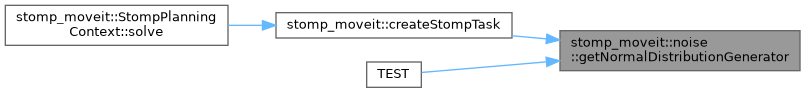moveit2 The MoveIt Motion Planning Framework for ROS 2.
stomp_moveit::noise Namespace Reference

## Functions

NoiseGeneratorFn getNormalDistributionGenerator (size_t num_timesteps, const std::vector< double > &stddev)

## ◆ getNormalDistributionGenerator()

 NoiseGeneratorFn stomp_moveit::noise::getNormalDistributionGenerator ( size_t num_timesteps, const std::vector< double > & stddev )

Creates a noise generator function that applies noise sampled from a normal distribution. The noise is applied over a matrix of size (num_timesteps, stddev.size()) which corresponds to the matrix representation of a robot trajectory.

Parameters
 num_timesteps the waypoint count of the trajectory stddev the standard deviation for each variable dimension (number of joints)

Definition at line 58 of file noise_generators.hpp.

Here is the caller graph for this function: